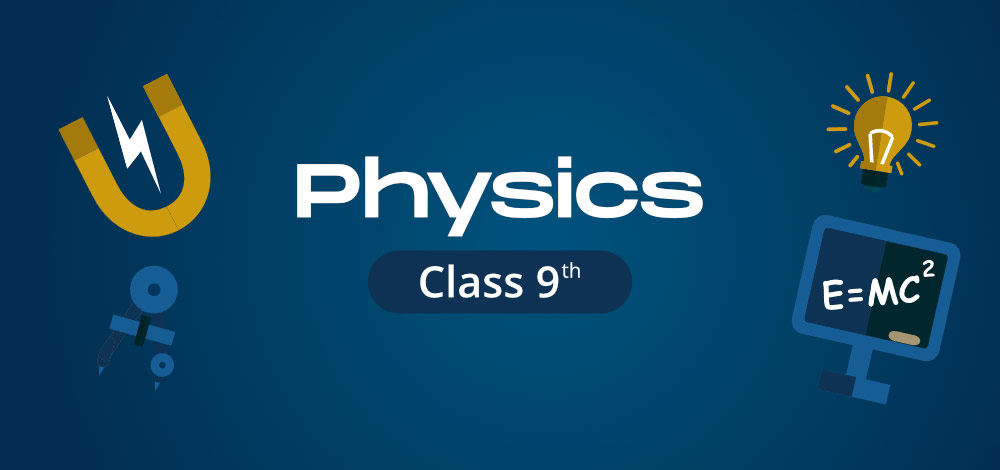GeeksforGeeks App
Open AppBrowser
Continue

# CBSE Class 9 Physics Notes

CBSE Class 9 Physics notes, GeeksforGeeks has created a new approach to school learning. Physics has been among the crucial subjects of the Class 9 Science Syllabus. It helps students in improving their understanding of fascinating concepts. The understanding of how motion, force, work, sound, gravity, energy, and power interact with one another and impact our lives. GeeksforGeeks prepared in-depth subject theory to have a solid foundation of Class 9 Physics. The below-listed articles are designed in such a way that you study everything according to your NCERT textbook, or reference books.### Chapter 1: Motion

The first chapter in the Physics curriculum of Class 9 is Motion. Motion means movement. Motion is defined as the change in position of an object with respect to its surrounding in a given interval of time. This chapter forms the basic idea of kinematics for students to learn in further classes. Therefore, CBSE Class 9 Physics notes elaborate on distance and displacement, uniform and non-uniform motion, speed, velocity, and accelerated and decelerated motion. It also explains the equation of motion with a graphical representation of motion and uniform circular motion as listed below:

### Chapter 2: Force and Laws of Motion

Force and Laws of Motion is the second chapter in the Class 9 Physics Syllabus. These notes explain the concept of force, its effects, balanced and unbalanced force, laws of motion, and Newton’s laws of motion. It further offers a detailed explanation of mass and inertia, momentum and mass, its unit along with numerical problems for practice. The notes also explain the second law of motion and the third law of motion. Below are the listed articles on Force and Laws of Motion:

### Chapter 3: Gravitation

The third chapter of the Physics in Science syllabus of Class 9 is Gravitation. Gravitation is the universal force of attraction acting between all matter. GeeksforGeeks crucial notes explain Newton’s universal law of gravitation, mass, weight, and the difference between mass and weight. The notes further provide solved numerical problems, which are discussed in the articles listed below:

### Chapter 4: Work and Energy

The Work and Energy is the fourth chapter in the Physics syllabus and so an important chapter to understand to solve mechanics in further classes. The notes talk about work, a condition when the work should be and when not, its unit, along with positive, negative, and zero work. The notes also elaborate on energy, its types, the law of conservation energy, power, a commercial unit of power and provide solved numericals, discussed in the articles mentioned below:

### Chapter 5: Sound

The last chapter in the Class 9 Physics Syllabus is Sound. Sound is heard every day from various sources like humans, birds, bells, machines, TV, radios, etc. The notes elaborate production and propagation of sound, sound waves as longitudinal waves, their characteristics, wavelength, frequency, time period, amplitude, and velocity. Various other concepts of the speed of sound in multiple mediums, sonic boom, echo, a reflection of sound, range of hearing, application of ultrasound, sonar, and structure of the human ear are also explained in the article listed below:

My Personal Notes arrow_drop_up
Related Tutorials